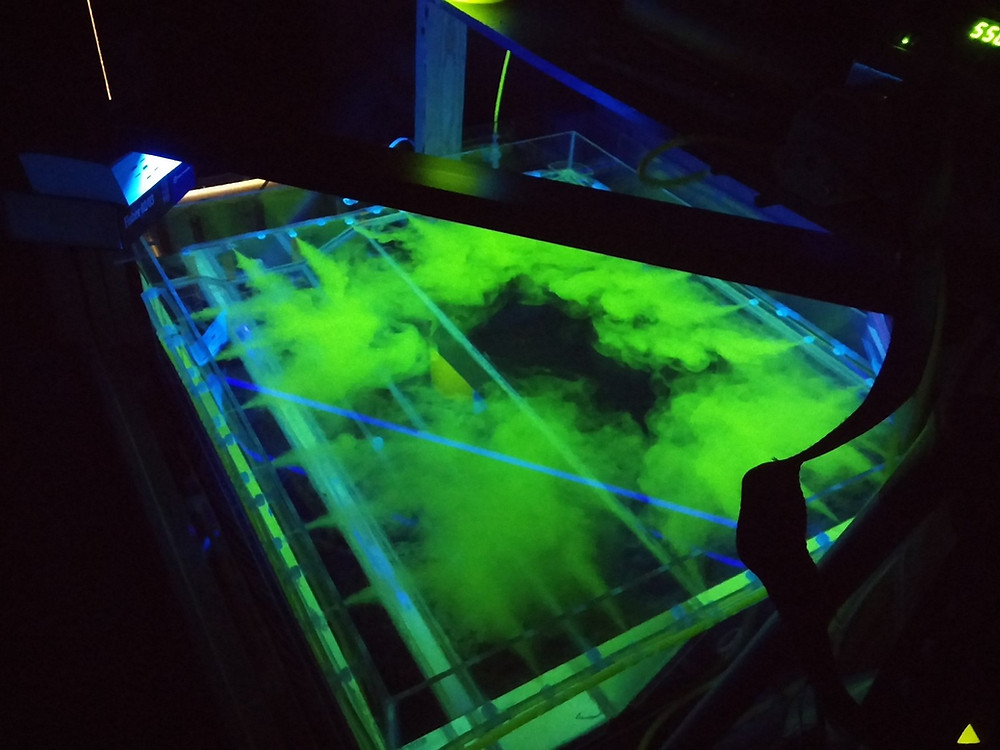•Amir Alansari

# Treatment Efficiency CalculatorThe work presented here is from a study done by Nate Madding to understand and quantify swimming pool re-circulation efficiency and its implications on treatment system operations. A 1:25 bench-scale swimming pool was constructed and salt tracer studies were performed in order to determine the effects of 1) turn-over rate, 2) inlet/outlet configuration, and 3) extent of mixing. The turnover rate refers to the time it takes for the entire volume of the pool to pass the treatment system. The results of the study were recently published in the Journal of Water and Health in an article titled: "A Quantitative Analysis of Swimming Pool Recirculation System Efficiency".

In summary, we found that the removal of salt tracer from the system could not be significantly altered by changing the system turnover rate, inlet/outlet configuration, or extent of mixing. The removal of salt from the system could be modeled using a simple exponential decay model with the decay constant being approximately equal to the inverse of the theoretical detention time, i.e. system volume divided by system flow rate. In other words, only 63% of the salt could be removed in a single turnover, 86% after a second turnover, and 95% after a third.

This implies that the overall treatment efficiency is limited by the percentage of contaminant delivered to the treatment system and not by the treatment system itself.

The time required to achieve a certain removal percentage of contaminant (based on the turnover rate and treatment system efficiency) can be calculated by using the following equation:Suppose a 50,000 [gal] swimming pool with a 25% efficient filter and a 6-hour turnover rate is contaminated with 1E+09 Cryptosporidium oocysts. After one turnover, the number of oocysts in the system will be approximately 7.78E+08 oocycts. In other words, only about 22% of the oocysts will be removed from the pool system after the first turnover (by removing 25% of the Cryptosporidium from 63% of the water). It would take approximately 28 turnovers (7 days) to reduce the total Cryptosporidium counts by 99.9%. For practical reasons, operators would be compelled to achieve their treatment goals in the shortest time possible. The figure below compares the time required to reduce a contaminants concentration by 99.9% (3-log) by changing the treatment efficiency and turnover rate.For a given treatment efficiency, reducing the turnover rate by a factor of 3 (6 to 2 hours) reduces the time required by the same factor. However, since reducing the turnover rate increases the flow rate in the system, the total volume of water required would remain the same. Similarly, the time required to achieve a 99.9% reduction decreases by the same factor as the treatment efficiency increases for a given turnover rate. Since the reduction in time occurs without a change in the turnover rate, the total volume required would also decrease by the same factor. This implies that it would be more practical for a swimming pool with a 25% efficient treatment system to improve the efficiency of their treatment system as opposed to reducing their turnover rate in order to reduce the total time and volume required to achieve their desired treatment levels. However, there is no practical benefit in improving the treatment efficiency beyond 90% since the total time and volume reduction would be insignificant. Instead, systems with greater than 90% treatment efficiency should reduce the turnover rate to reduce the required time.

I put together a simple excel macro that would calculate the time required to achieve a desired contaminant reduction level for a given system. Download Link